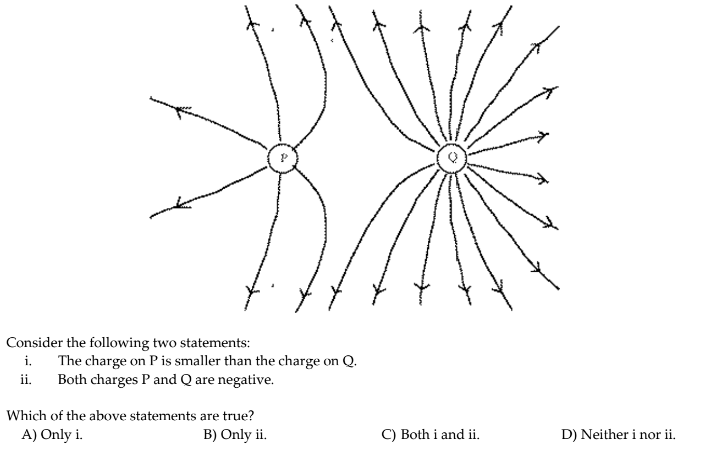Problem: The Figure below shows electric field lines arising from two small charged particles P and Q.

FREE Expert Solution
82% (482 ratings)
Problem Details

The Figure below shows electric field lines arising from two small charged particles P and Q.What scientific concept do you need to know in order to solve this problem?

Our tutors have indicated that to solve this problem you will need to apply the Electric Field Lines concept. You can view video lessons to learn Electric Field Lines Or if you need more Electric Field Lines practice, you can also practice Electric Field Lines practice problems .

What is the difficulty of this problem?

Our tutors rated the difficulty of The Figure below shows electric field lines arising from two... as low difficulty.

How long does this problem take to solve?

Our expert Physics tutor, Douglas took 4 minutes to solve this problem. You can follow their steps in the video explanation above.

What professor is this problem relevant for?

Based on our data, we think this problem is relevant for Professor Evans' class at GSU.